Courses

# Test: Single Correct MCQs: Differentiation | JEE Advanced

## 11 Questions MCQ Test Mathematics For JEE | Test: Single Correct MCQs: Differentiation | JEE Advanced

Description
This mock test of Test: Single Correct MCQs: Differentiation | JEE Advanced for JEE helps you for every JEE entrance exam. This contains 11 Multiple Choice Questions for JEE Test: Single Correct MCQs: Differentiation | JEE Advanced (mcq) to study with solutions a complete question bank. The solved questions answers in this Test: Single Correct MCQs: Differentiation | JEE Advanced quiz give you a good mix of easy questions and tough questions. JEE students definitely take this Test: Single Correct MCQs: Differentiation | JEE Advanced exercise for a better result in the exam. You can find other Test: Single Correct MCQs: Differentiation | JEE Advanced extra questions, long questions & short questions for JEE on EduRev as well by searching above.
QUESTION: 1

### If y2 = P(x) , a polynomial of degree 3, then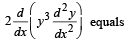Solution:

We have  y2 = P (x), ... (1)
where P(x) is a polynomial of degree 3 and hence thrice differentiable. Differentiating (1) w.r. to x, we get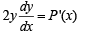......(2)

Again differentiating with respect to x, we get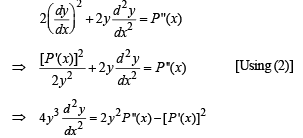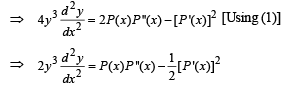Again differentiating w.r. to x, we get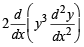= P '''(x) P (x) + P ''(x) P '(x)- P '(x) P ''(x)
= P '''(x) P(x)

QUESTION: 2

### Let f (x) be a quadratic expression which is positive for all the real values of x. If g(x) = f (x) + f '(x) + f ''(x), then for any real x,

Solution:

Let f (x) = ax2 + bx + c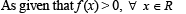∴ a > 0 and D < 0
⇒ a > 0 and b2 – 4ac < 0 ..... (1)
Now, g (x) = f (x) + f ' (x) + f '' (x)
= ax2 + bx + c + 2ax + b + 2a
= ax2 + (2a + b) x + (2a + b + c)
Here, D = (2a + b)2 – 4a(2a + b + c)
= 4a2 + b2 + 4ab – 8a2 – 4ab – 4ac
= b2 – 4a2 – 4ac = – 4a2 + b2 – 4ac
= (–ve) + (–ve) = –ve [Using eq. (1)] Also a > 0 from (1)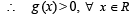QUESTION: 3

###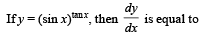Solution:

y = (sin x) tan x ⇒ log y= tan x. log sinx
Differentiating w.r.t. x,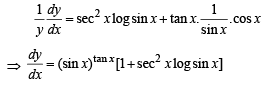QUESTION: 4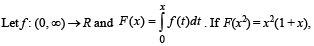then f(4) equals

Solution: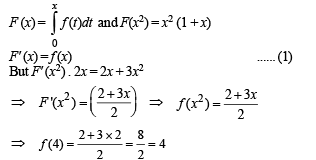QUESTION: 5

If x2 + y2 = 1 then

Solution:

x2 + y2 = 1

⇒ 2x + 2yy' = 0

⇒ x + yy'= 0
⇒ 1+ yy'' + (y')2 = 0

⇒ yy'' + (y')2+1 = 0

QUESTION: 6

If y is a function of x and log (x + y) – 2xy = 0, then the value of y' (0) is equal to

Solution:

log (x + y) = 2xy when x = 0 then y = 1
Differentiating w.r.t. x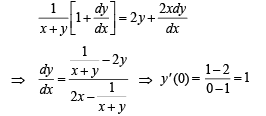QUESTION: 7

If f(x) is a twice differentiable function and given that f(1) = 1; f(2) = 4, f(3) = 9, then

Solution:

Let us  consider the function g (x) = f (x) – x2  so that
g (1) = f (1) – 12 = 1 – 1 = 0
g (2) = f (2) – 22 = 4 – 4 = 0
g (3) = f (3) – 32 = 9 – 9 = 0
Since f (x) is twice differentiable we can say g (x) is continuous and differentiable everywhere and
g (1) = g (2) = g (3) = 0
∴ By Rolle's theorem, g' (c) = 0 for some c ∈ (1,2)
and g '(d) =0 for some d ∈ (2,3)
Again by Rolle's theorem,
g ''(e) =0 for some e ∈ (c,d) ⇒ e ∈ (1,3)
⇒ f ''(e) - 2 = 0 or f ''(e) = 2 for some x ∈ (1, 3)
f ''(x) = 2 for some x∈ (1,3)

QUESTION: 8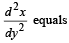Solution: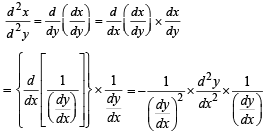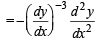QUESTION: 9

Let g (x) = log f (x) where f (x) is  twice differentible positive function on (0, ∞) such that f (x + 1) = x f (x). Then, for N = 1, 2, 3, ...........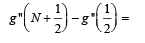Solution:

Given that g(x) = log f (x) ⇒ g (x + 1) = log f (x + 1)
⇒ g (x + 1) = log x f (x)  [∴ f (x + 1) = x f (x)]
⇒ g(x + 1) = logx + log f (x) ⇒ g(x + 1) – g(x) = log(x)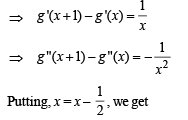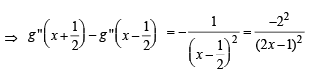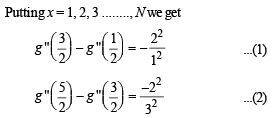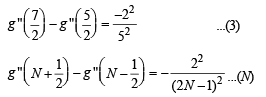Adding all the above equations, we get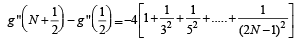QUESTION: 10

Let f : [0, 2] → R be a function which is continuous on [0, 2] and is differentiable on (0, 2) with f (0) = 1. Let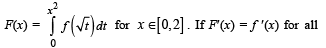x ∈ (0,2) , then F(2) equals

Solution: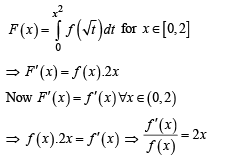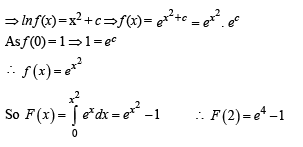*Multiple options can be correct
QUESTION: 11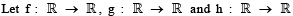be differentiable functions such that f(x) = x3 + 3x + 2, g(f(x)) = x and h (g(g(x))) = x for all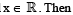Solution:

f(x) = x3 + 3x + 2
⇒ f '(x) = 3x2 + 3
Also
f(0) = 2,
f (1) = 6,
f(2) = 16,
f(3) = 38,
f(6) = 236
And
g(f(x)) = x
⇒ g(2) = 0,
g(6) = 1,
g(16) = 2,
g (38) = 3,
g (236) = 6

(a) g (f(x)) = x
⇒ g' (f(x)). f ¢(x) = 1
For
g'(2), f(x) = 2
⇒ x = 0
∴ Putting x = 0,
we get g'(f (0)) f '(0) = 1
⇒ g'(2) = 1/3

(b) h (g(g(x))) = x
⇒ h'(g(g(x))). g'(g(x)). g'(x) = 1 For h'(1),
we need g (g(x)) = 1
⇒ g (x) = 6
⇒x = 236
∴ Putting x = 236, we get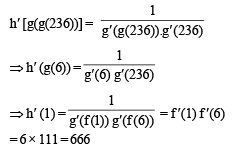(c) h [g(g(x))] = x
For h (0), g (g(x)) = 0
⇒ g(x) = 2
⇒ x = 16
∴ Putting x = 16, we get
h(g(g(16))) = 16
⇒ h(0) = 16

(d) h[g(g(x))] = x
For h(g(3)), we need g(x) = 3
⇒ x = 38
∴ Putting x = 38,
we get
h [g(g(38))] = 38
⇒ h (g(3)) = 38